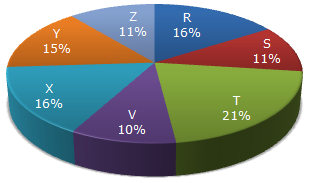# Data Interpretation - Pie Charts - Discussion

Study the following pie-chart and the table and answer the questions based on them.

Proportion of Population of Seven Villages in 1997Village % Population Below Poverty Line X 38 Y 52 Z 42 R 51 S 49 T 46 V 58

3.

Find the population of village S if the population of village X below poverty line in 1997 is 12160.

 [A]. 18500 [B]. 20500 [C]. 22000 [D]. 26000

Explanation:

Let the population of village X be x.

 Then, 38% of x = 12160x = 12160 x 100 = 32000. 38

Now, if s be the population of village S, then

 16 : 11 = 32000 : ss =11 x 3200= 22000. 16

 Archana Mirashe said: (Jun 13, 2014) How does 16 : 11 Has come I don't understand?

 Hina said: (Jul 16, 2014) How does 16 : 11 Has come I don't understand?

 Kasinath @Hyd said: (Aug 21, 2014) 16:11 means percentage of X and percentage of S. 16% X =32000. then 11% S be y. y = (32000*11)/16 = 22000.

 Vinay Singh said: (Feb 2, 2018) Instead, you can just calculate the total population and arrive at population simply. The question contains unnecessary information! TOTAL POPULATION=200000 ;S=200000*11/100=22000.